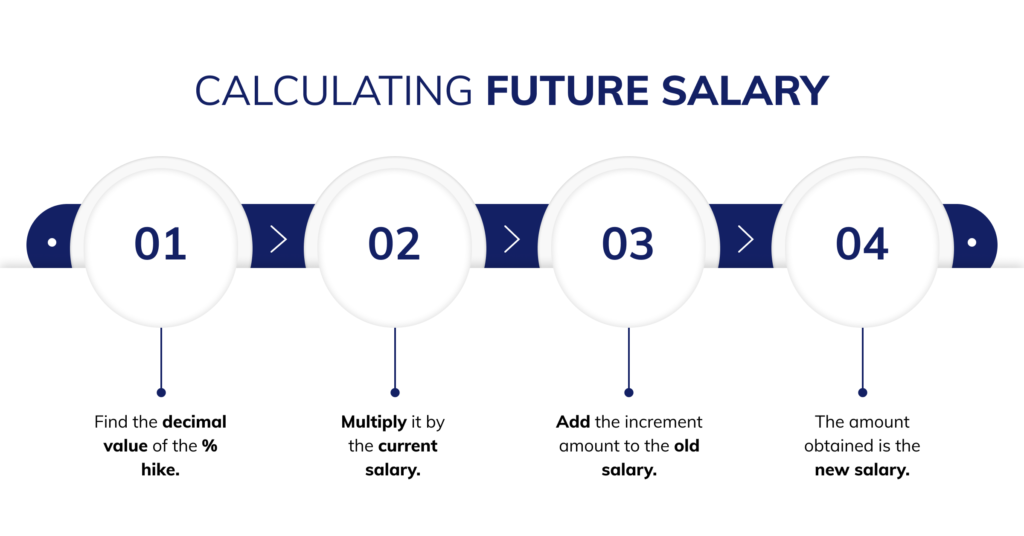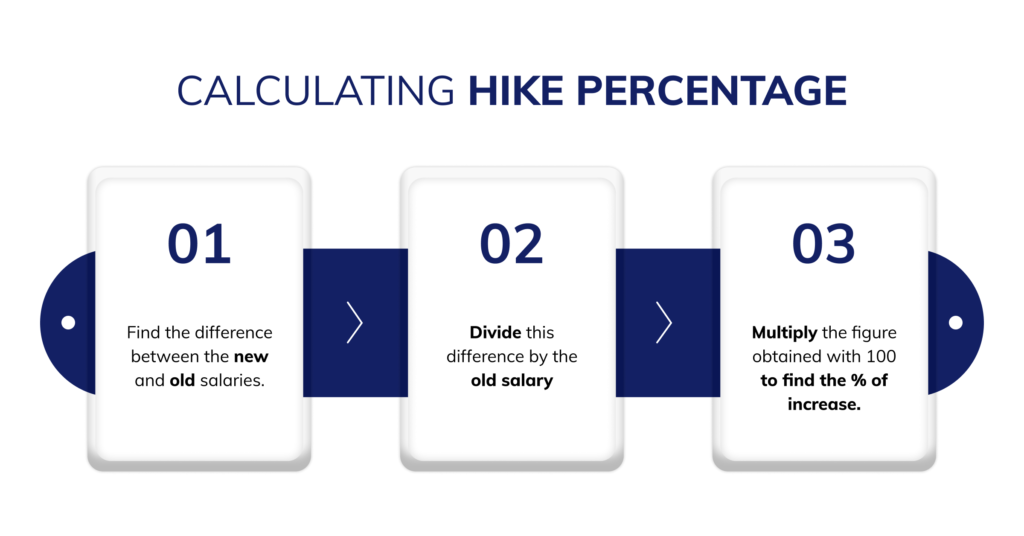An increment or a salary hike is the increase in the current salary of any employee by a certain amount. It is expressed as a percentage of the current salary. Increments can be given quarterly, half-yearly, or yearly and are also used by employees to negotiate their salaries with a new employer.

Calculating the salary hike percentage is very important for both the employer and the employee. If you don’t know how to calculate salary hike percentage in India, you’ve landed on the perfect article.

## How to calculate salary hike percentage?

Salary hikes can be either expressed as a percentage or absolute amount.

There are two ways to calculate the salary hike percentage:Let us look at an example to understand more.

### An example of how to calculate the percentage of hike in salary

Let’s say that the current salary of Shubham is INR 30,000. The company is giving him a hike of 40% on his current salary. Then the future salary will be calculated as follows –

 Current Salary (in INR) Hike Percentage Formula Future Salary/ Salary after Increment (in INR) 30,000 40% 30,000*40% = 12000 (30000+12000) Total Future Salary 42000

Let us take forward the example given above. Let’s say the current salary of Mr X is INR 30,000 and his future salary after increment is INR 42,000. Then the percentage of hike in salary will be calculated as follows –

 Current Salary (in INR) Future Salary/ Salary after Increment (in INR) Formula* Percentage Hike 30000 42000 (42000-30000)/30000*100 0.4*100 Percentage Hike in Salary 40%

Formula for calculating percentage hike in salary is : (New Salary – Old Salary)/Old Salary*100

### Skip the hassle of manual calculation with RazorpayX Payroll

Now you can calculate payroll and salary hikes with just 3 clicks with RazorpayX Payroll. RazorpayX Payroll provides automatic salary calculation after considering the statutory deductions and compliances like PF, PT, TDS & ESIC.

Here are the other benefits of RazorpayX Payroll –

• End-to-end employee management with offer letter generation and CTC calculator
• Automated salary deductions based on statutory compliances
• Automated group health insurance
• Comprehensive leave management system

## FAQs

### 1. How is the hike percentage calculated?

The salary hike percentage is calculated using the following formula –

(New salary – Old Salary) / Old Salary * 100 = Hike percentage.

• The first step is to find the difference between the new salary and the old salary
• Then the amount obtained is divided by the old salary.
• The figure thus obtained is multiplied by 100 to express it in percentage.

### 2. How is a 30 per cent hike in salary calculated?

A 30 % hike in salary is calculated as follows –

For better understanding, let’s assume the current salary to be INR 10,000

 Current Salary (in INR) Hike Percentage Formula Future Salary/ Salary after Increment (in INR) 10000 30% 10000*30% = 3000 (10000+3000) Total Future Salary 13000Alish is a writer at RazorpayX. Zoophilist. Coffee Addict. And now a FinTech enthusiast. When not writing you'll find her learning French, taking a long walk (or run) with her dog, or Netflixing.

Disclaimer: Banking Services and Razorpay powered Current Account is provided by Scheduled Banks